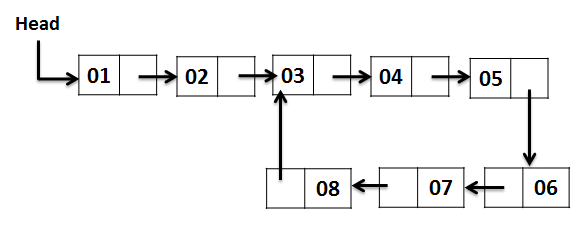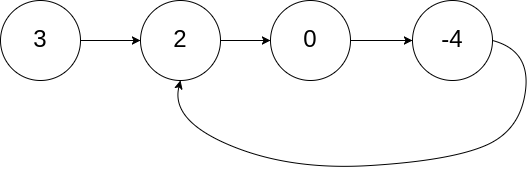## Find the start point of a Loop in a Singly LinkedList if there is any Loop?

In this article we are going to find the node in a singly `LinkedList`, where a Loop starts, if there is a loop that exists in the LinkedList. The solution is an extension to the problem of finding the existence of a Loop in a linked list. Finding the loop in a linked list was solved using Floyd cycle detection algorithm in this article. It is highly recommended to read the cycle finding solution before continuing in this solution. Consider the following figure of a singly linked list with a loop where the starting position of the loop is the third node with value 3.After finding the loop in the linked list, we initialize the `slowPtr` to the head of the linked list. From that point onwards both `slowPtr` and `fastPtr` move only one node at a time. The point at which they meet is the start of the loop. Generally, we use this method for removing the loop. Following is the linked list ADT we are using for solving the problem.

``````//Definition for singly-linked list.
class ListNode {
int val;
ListNode next;
ListNode(int x) {
val = x;
next = null;
}
}``````

Example 1:

```Input: head = [3,2,0,-4], pos = 1
Output: tail connects to node index 1```The following method will return the `Node`, which is the starting position of the loop, or returns NULL if there is no loop present in the singly linked list.

``````/**
* class ListNode {
*     int val;
*     ListNode next;
*     ListNode(int x) {
*         val = x;
*         next = null;
*     }
* }
*/
public class Solution {
if (head == null) return null;
if (head.next == null) return null;
boolean cycleFound = false;

// A fast pointer will either loop around a cycle and meet the slow
// pointer or reach the `null` at the end of a non-cyclic list.
while (fastPtr != null && fastPtr.next != null) {
fastPtr = fastPtr.next.next;
slowPtr = slowPtr.next;
if (slowPtr == fastPtr) {
cycleFound = true;
break;
}
}

// To find the entrance to the cycle, we have two pointers traverse at
// the same speed -- one from the front of the list, and the other from
// the point of intersection.
ListNode loopStartAt = null;
if (cycleFound) {
while (true) {
if (slowPtr == fastPtr) {
loopStartAt = slowPtr;
break;
}
slowPtr = slowPtr.next;
fastPtr = fastPtr.next;
}
}
return loopStartAt;
}
}``````